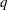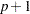The CATMOD Procedure
 Output Data Sets

### OUT= Data Set

For each population, the OUT= data set contains the observed and predicted values of the response functions, their standard errors, the residuals, and variables that describe the population and response profiles. In addition, if you use the standard response functions, the data set includes observed and predicted values for the cell frequencies or the cell probabilities, together with their standard errors and residuals.

#### Number of Observations

For the standard response functions, there areobservations in the data set for each BY group, whereis the number of populations andis the number of response functions per population. Otherwise, there areobservations in the data set for each BY group.

#### Variables in the OUT= Data Set

The data set contains the following variables:

BY variables

If you use a BY statement, the BY variables are included in the OUT= data set.

dependent variables

If the response functions are the default ones (generalized logits), then the dependent variables, which describe the response profiles, are included in the OUT= data set. When _TYPE_=FUNCTION, the values of these variables are missing.

independent variables

The independent variables, which describe the population profiles, are included in the OUT= data set.

_NUMBER_

the sequence number of the response function or the cell probability or the cell frequency

_OBS_

the observed value

_PRED_

the predicted value

_RESID_

the residual (observed minus predicted)

_SAMPLE_

the population number. This matches the sample number in the "Population Profile" section of the output.

_SEOBS_

the standard error of the observed value

_SEPRED_

the standard error of the predicted value

_TYPE_

specifies a character variable with three possible values. When _TYPE_=FUNCTION, the observed and predicted values are values of the response functions. When _TYPE_=PROB, they are values of the cell probabilities. When _TYPE_=FREQ, they are values of the cell frequencies. Cell probabilities or frequencies are provided only when the default response functions are modeled. In this case, cell probabilities are provided by default, and cell frequencies are provided if you specify the option PRED=FREQ.

### OUTEST= Data Set

This TYPE=EST output data set contains the estimated parameter vector and its estimated covariance matrix. If you specify both the ML and WLS options in the MODEL statement, the OUTEST= data set contains both sets of estimates. For each BY group, there areobservations in the data set for each estimation method, whereis the number of estimated parameters. The data set contains the following variables:

B1, B2, and so on

variables for the estimated parameters. The OUTEST= data set contains one variable for each estimated parameter.

BY variables

If you use a BY statement, the BY variables are included in the OUT= data set.

_METHOD_

the method used to obtain parameter estimates. For weighted least squares estimation, _METHOD_=WLS, and for maximum likelihood estimation, _METHOD_=ML.

_NAME_

identifies parameter names. When _TYPE_=PARMS, _NAME_ is blank, but when _TYPE_=COV, _NAME_ has one of the values B1, B2, and so on, corresponding to the parameter names.

_STATUS_

indicates whether the estimates have converged

_TYPE_

identifies the statistics contained in the variables for parameter estimates (B1, B2, and so on). When _TYPE_=PARMS, the variables contain parameter estimates; when _TYPE_=COV, they contain covariance estimates.

The variables _METHOD_, _NAME_, and _TYPE_ are character variables; the BY variables can be either character or numeric; and the variables for estimated parameters are numeric.

See Appendix A, Special SAS Data Sets, for more information about special SAS data sets.Previous Page | Next Page | Top of Page